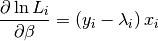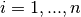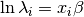# statsmodels.discrete.discrete_model.Poisson.jac¶

Poisson.jac(*args, **kwds)

jac is deprecated, use score_obs instead! Use score_obs method. jac will be removed in 0.7

Poisson model Jacobian of the log-likelihood for each observation
Parameters: params : array-like The parameters of the model score : ndarray (nobs, k_vars) The score vector of the model evaluated at params

Notesfor observationswhere the loglinear model is assumed